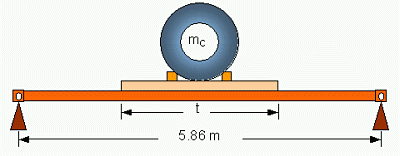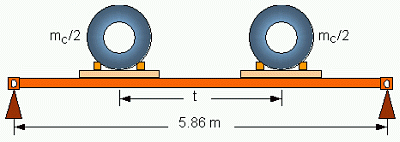3.2   Loading the container longitudinallyThe longitudinal distance of the centres of the corner fittings is 5.853 m. This figure is only little less than the usable inner length of 5.867 m, so that the calculation of the maximum bending moment is based on a length of the homogeneous load and an equal distance of supports of both 5.86 m.Figure 9: Shear forces and bending moments in the side walls from a homogeneous load The homogeneous loading of a container produces shear forces and bending moments in the side walls of a container as shown in Figure 9. The maximum bending moment from the load P can be calculated as:This expression serves as limiting value for inhomogeneous loads, which may appear when loading steel coils. If a coil of the mass mC is placed on longitudinal beams of the length t in the container (Figure 10), a bending moment results at half the length of the container of:Figure 10: Uniform load of length t longitudinally in a container This bending moment must not exceed the limiting value presented by equation (4). The mass mC must therefore be restricted to:This load case applies to usual bedding arrangements for coils of weight classes H and XH. If two coils of the same mass are placed longitudinally into a container as shown in Figure 11, the full payload of the container may only be utilised, if the coils are not stowed closer to each other than at ¼ and ¾ of the container length, so that the distance t is at least 2.93 m. For a distance less than 2.93 m, the mass of both coils has to be reduced to:Figure 11: Separated load longitudinally in a container If three coils of the same mass are placed longitudinally into a container, they have to be stowed at 1/7, 1/2 and 6/7 of the container length for utilising the full payload of the container. That means the centres of the end coils have a distance to the end walls of only 84 cm. If four coils of the same mass are placed longitudinally into a container, they have to be stowed at 1/8, 3/8, 5/8 and 7/8 of the container length for utilising the full payload of the container. That means the centres of the end coils have a distance to the end walls of only 73 cm.Contact  |  Site Map  |  Glossary  |  Legal Notice